# MATLAB help 75% ▼ Photoshoped Image Original Image 75% ▼ Photoshoped Image Original Image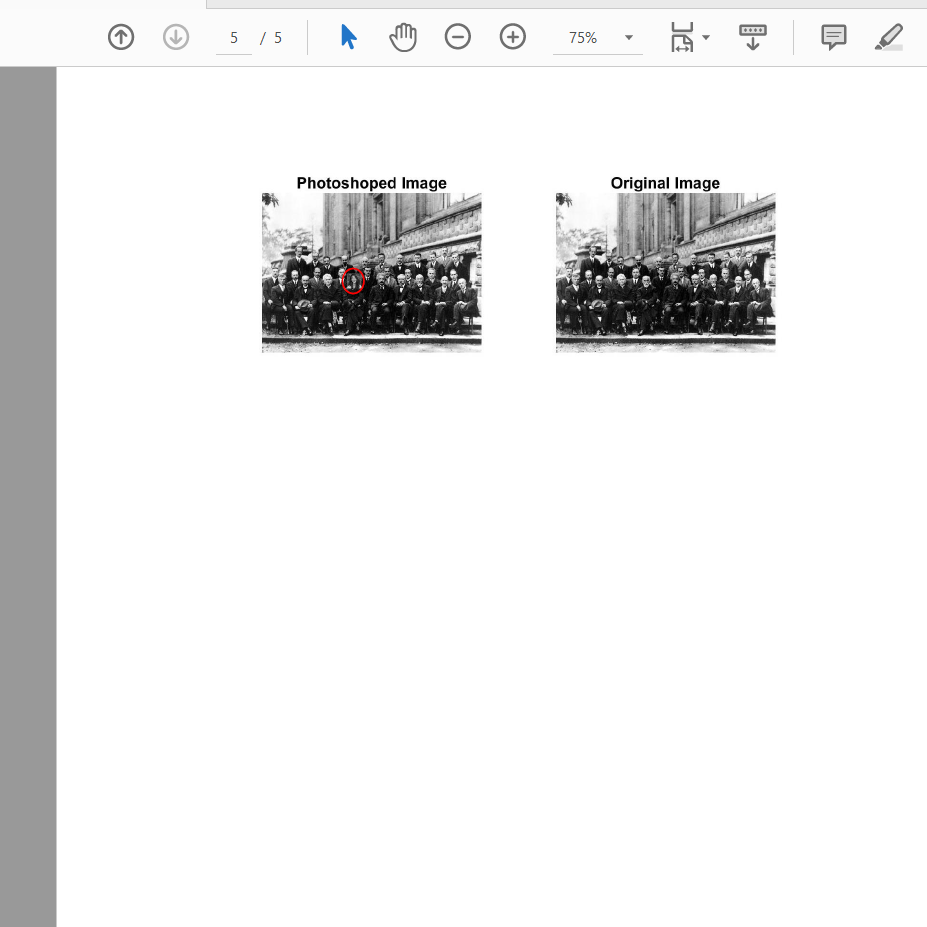MATLAB help

75% ▼ Photoshoped Image Original Image

startingFolder = pwd; % or 'C:\Program Files\MATLAB' or wherever...

if ~exist(startingFolder, 'dir')

% If that folder doesn't exist, just start in the current folder.

startingFolder = pwd;

end

% Get the name of the file that the user wants to use.

defaultFileName1 = fullfile(startingFolder, '*.*');

[baseFileName1, folder1] = uigetfile(defaultFileName1, 'Select Bicubic file');

defaultFileName2 = fullfile(startingFolder, '*.*');

[baseFileName2, folder2] = uigetfile(defaultFileName2, 'Select Other method file');

if baseFileName1 == 0

% User clicked the Cancel button.

return;

end

fullSourceFileName1 = fullfile(folder1, baseFileName1)

if baseFileName2 == 0

% User clicked the Cancel button.

return;

end

fullSourceFileName2 = fullfile(folder2, baseFileName2)

% Read images to be differenced from source file names

% Resize images

firstImageresize = (imresize(firstImage, [504, 504])); % Bicubic Method

secondImageresize = (imresize(secondImage, [504, 504])); % Methods to compare

% Image difference

difference_image = double(secondImageresize) - double(firstImageresize);

imshow(difference_image)

% Saving results

% Create destination filename

destinationFolder = 'C:/Set5 [X2]';

if ~exist(destinationFolder, 'dir')

mkdir(destinationFolder);

end

% Strip off extenstion from input file

[sourceFolder, baseFileNameNoExtenstion, ext] = fileparts(fullSourceFileName1);

% Create jpeg filename. Don't use jpeg format for image analysis!

outputBaseName = [baseFileNameNoExtenstion, '.JPG']

fullDestinationFileName = fullfile(destinationFolder, outputBaseName);

% Write the jpg file. This will convert whatever format you started with to the hated jpg format.

imwrite(difference_image, fullDestinationFileName);

##### Add Answer of: MATLAB help 75% ▼ Photoshoped Image Original Image 75% ▼ Photoshoped Image Original Image
Similar Homework Help Questions
• ### Matlab Exercise a)Filter the image using both filters and display the original image(By loading and displaying ‘rice.png...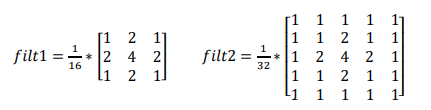Matlab Exercise a)Filter the image using both filters and display the original image(By loading and displaying ‘rice.png’ image by converting variable format to “double”.) and filtered images. (Image must be displayed in gray scale. Axis spacings must be equal and axis labels must be turned off. Each image must have its own color bar.) b). Take [256, 256] Fourier Transform of the image ,[256, 256] Fourier Transform of the filters and take inverse Fourier Transform of filtered images. b)What kind...

• ### I need help to find out the MATLAB code to solve this equation. Thanks By using MATLAB program, Find the Gradient of Vi = 24Vocos(Ty/3) sin (2T1/3) By using MATLAB program, Find the Gradient of...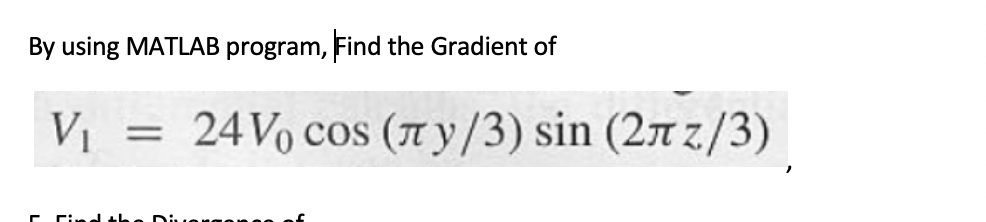I need help to find out the MATLAB code to solve this equation. Thanks By using MATLAB program, Find the Gradient of Vi = 24Vocos(Ty/3) sin (2T1/3) By using MATLAB program, Find the Gradient of Vi = 24Vocos(Ty/3) sin (2T1/3)

• ### 2/11/20 EN T G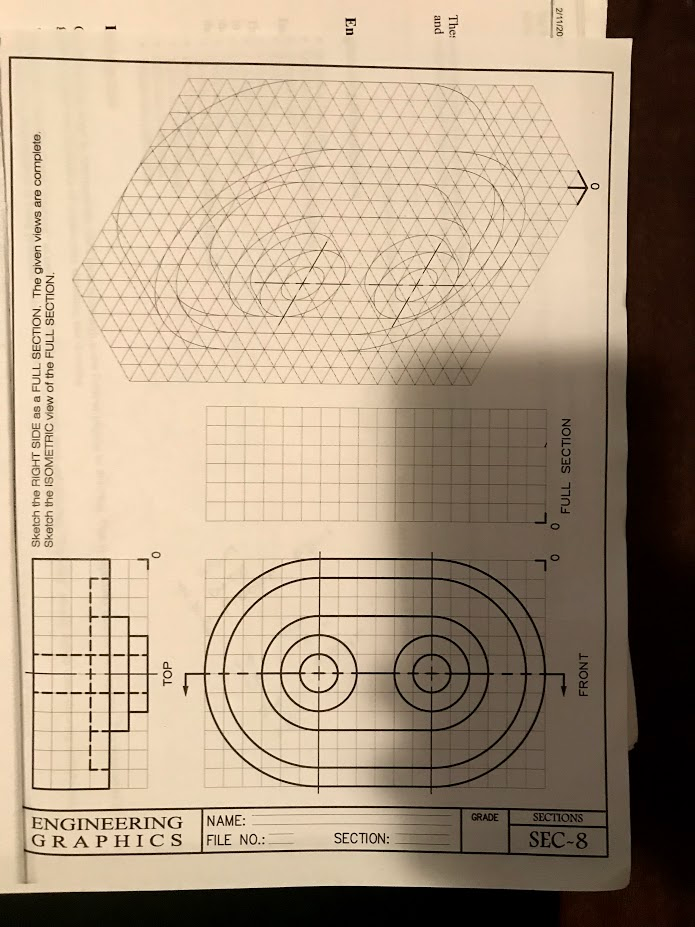2/11/20 EN T G 2/11/20 EN T G

• ### Perform Zooming to the particular portion of the image through figure window in Matlab. Specify each program lines of Matlab with appropriate comments.(25 Marks) 4. Perform Zooming to the partic...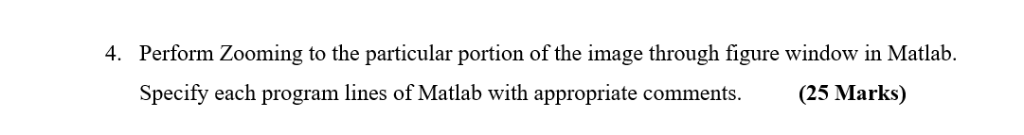Perform Zooming to the particular portion of the image through figure window in Matlab. Specify each program lines of Matlab with appropriate comments.(25 Marks) 4. Perform Zooming to the particular portion of the image through figure window in Matlab. Specify each program lines of Matlab with appropriate comments.(25 Marks) 4.

• ### .y 0.7in75 kips 16 ft W8 x 31 75 kips Part 1 out of 2 in. 1m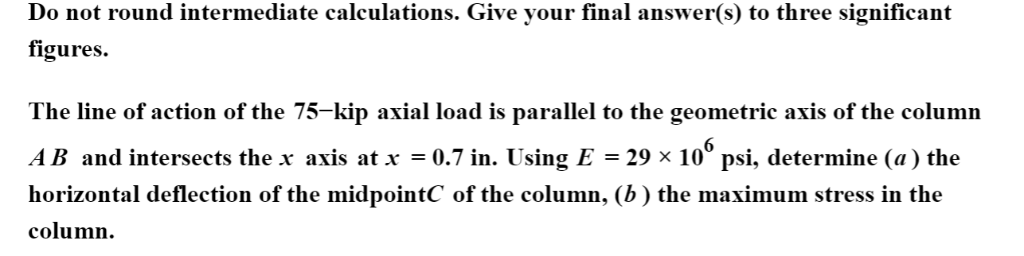We were unable to transcribe this image.y 0.7in75 kips 16 ft W8 x 31 75 kips Part 1 out of 2 in. 1m .y 0.7in75 kips 16 ft W8 x 31 75 kips Part 1 out of 2 in. 1m

• ### So I did 4 and 5 and got 12/5 and 1/9 respectively. But I need help with doing number 6, thanks. Evaluate. 3 (4 5 4. 6....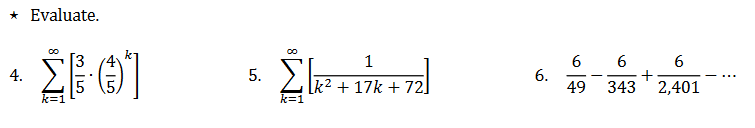So I did 4 and 5 and got 12/5 and 1/9 respectively. But I need help with doing number 6, thanks. Evaluate. 3 (4 5 4. 6. 49 343 2,401 k2 +17k +72 5 15 Evaluate. 3 (4 5 4. 6. 49 343 2,401 k2 +17k +72 5 15

• ### nJ ·IA the ร Show transcribed image text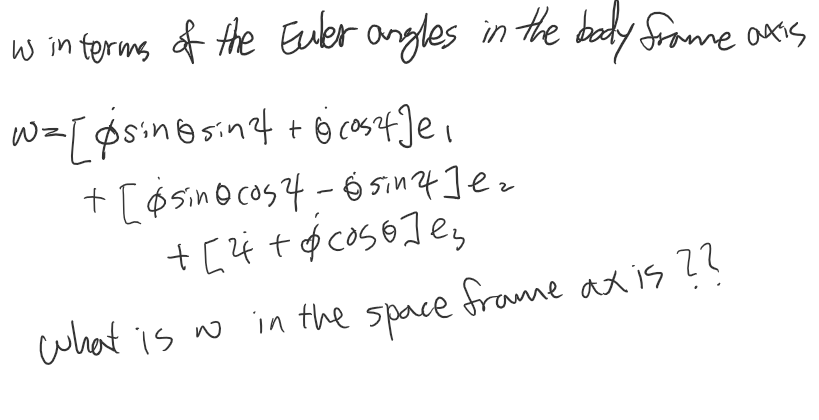nJ ·IA the ร Show transcribed image text

• ### slider P Y-75 mls 125 mm 65 mm A. 40mm slider P Y-75 mls 125 mm 65 mm A. 40mm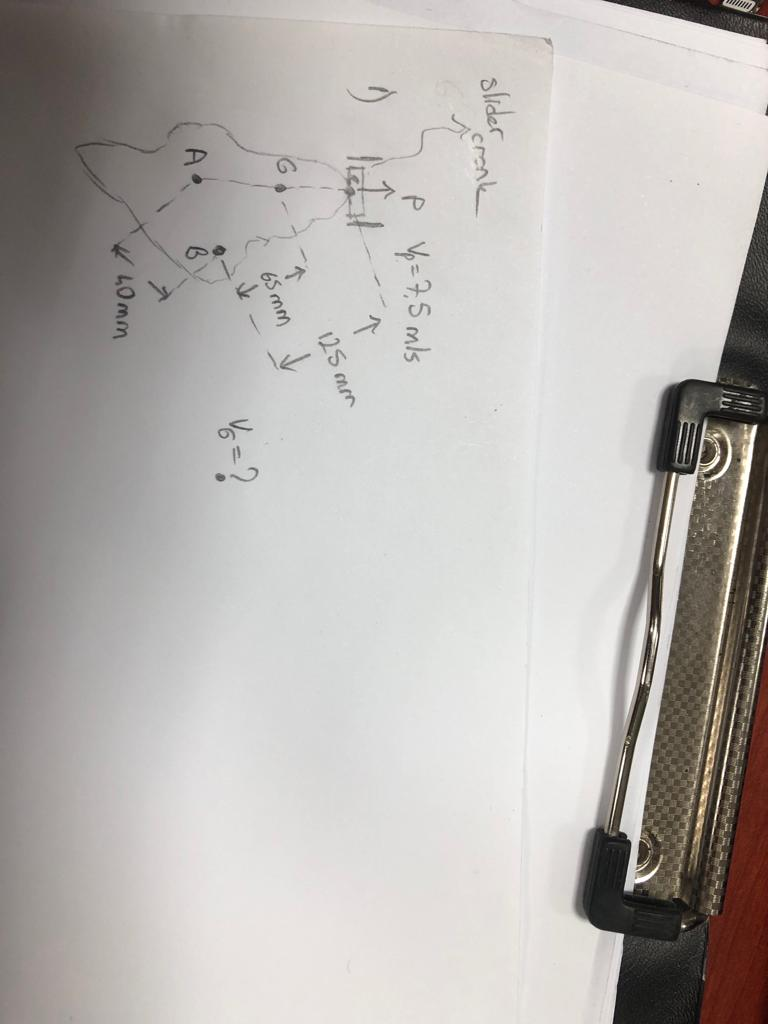slider P Y-75 mls 125 mm 65 mm A. 40mm slider P Y-75 mls 125 mm 65 mm A. 40mm

• ### Hi can you help me solve the following Signals and System (Continuous Time Fourier Series) question using MATLAB and show me the code for the MATLAB. 1. Using MATLAB graph each sum of complex sinusoi...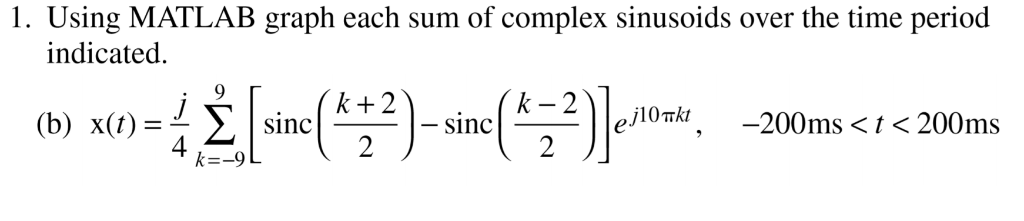Hi can you help me solve the following Signals and System (Continuous Time Fourier Series) question using MATLAB and show me the code for the MATLAB. 1. Using MATLAB graph each sum of complex sinusoids over the time period indicated. 9 r k-2 に-9L 1. Using MATLAB graph each sum of complex sinusoids over the time period indicated. 9 r k-2 に-9L

• ### PLease urgent help me.(Singular Value Decomposition-MATLAB) THE SVD THEOREM Develop a one line MATLAB command to comput...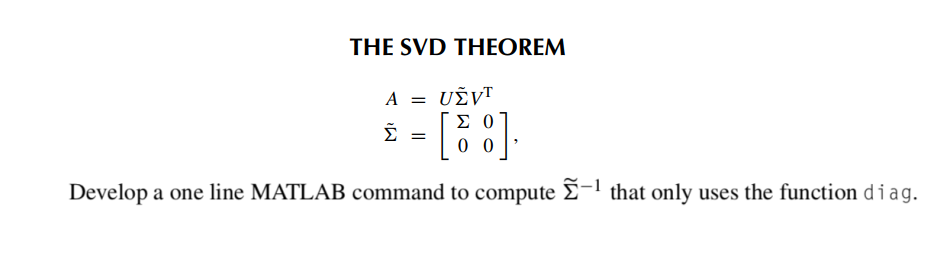PLease urgent help me.(Singular Value Decomposition-MATLAB) THE SVD THEOREM Develop a one line MATLAB command to compute Σ-1 that only uses the function d i a g. THE SVD THEOREM Develop a one line MATLAB command to compute Σ-1 that only uses the function d i a g.

Need Online Homework Help?